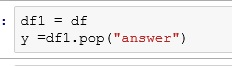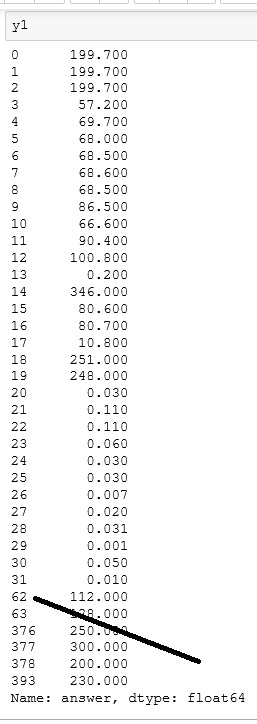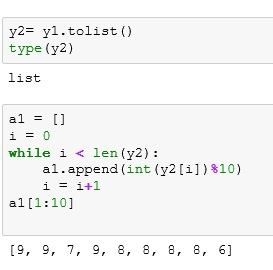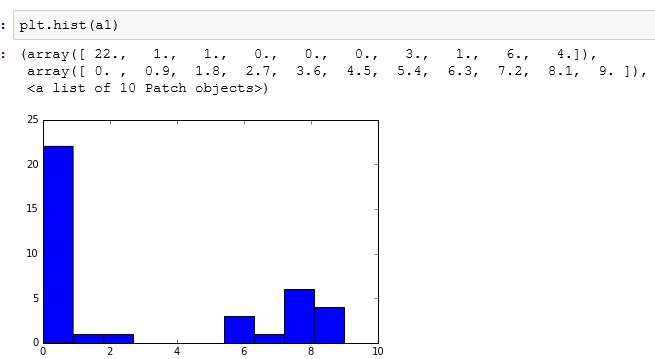# Python: An Interesting Problem with Pandas

I was writing a little tongue and cheek article for LinkedIn on fraud detection using frequency distributions (you can read the article here: LinkedIn). While this was a non-technical article, I wanted to use some histograms from a real data set, so I uploaded a spread sheet into Python and went to work.

While working with the data I ran into an interesting problem that had me chasing my tail for about 10 minutes before I figured it out. It is a fun little problem involving Series and Dataframes.

As always, you can upload the data set here: FraudCheck1

```import pandas as pd
("C:\\Users\\Benjamin\\OneDrive\\Documents\\article\\python\\FraudCheck1.xlsx")The data is pretty simple here. We are concerned with our answer column and the CreatedBy (which is the employee ID).  What I am trying to do is see if the “answer”  (a reading from an electric meter) are really random or if they have been contrived by someone trying to fake the data.

First, I want to get the readings for all the employees, so I used pop() to place the answer column into a separate list.

```df1 = dfThen, to make my histogram more pleasant looking, I decided to only use the last digit before the decimal. That way I will have 10 bars (0-9). (Remember, this is solely for making charts for an article. So I was not concerned with any more stringent methods of normalization)

What I am doing below is int(199.7%10). Remember % is the modulus – leaves you with the remainder and int converts your float to an integers. So 199.7 is cut to 199. The 199/10 remainder = 9.

```a= []
i = 0
while i < len(y):
a.append(int(y[i]%10))
i += 1
a[1:10]```Then I created my histogram.

```%matplotlib inline
from matplotlib import pyplot as plt
plt.hist(a)```## Now my problem

Now I want graph only the answers from employee 619, so first I filter out all rows but the ones for employee 619.

```df2 = df.query('CreatedBy == 619')

Then I ran my loop to turn my answers into a single digit.

And I get an error.  Why?Well the answer lies in the datatypes we are working with. Pandas read_excel function creates a Dataframe.

When you pop a column from a dataframe, you end up with a Series. And remember a series is an indexed listing of values.

Let’s look at our Series. Check out the point my line is pointing to below. Notice how my index jumps from 31 to 62. My while loop counts by 1, so after 31, I went looking for y1 and it doesn’t exist.Using .tolist() converts our Series to a list and now our while loop works.And now we can build another histogram.## The Code

```import pandas as pd
("C:\\Users\\Benjamin\\OneDrive\\Documents\\article\\python\\FraudCheck1.xlsx")

df1 = df

a= []
i = 0
while i < len(y):
a.append(int(y[i]%10))
i += 1
a[1:10]

%matplotlib inline
from matplotlib import pyplot as plta1 = []
i = 0
while i < len(y2):
a1.append(int(y2[i])%10)
i = i+1
a1[1:10]

plt.hist(a)

df2 = df.query('CreatedBy == 619')

y2= y1.tolist()
type(y2)

a1 = []
i = 0
while i < len(y2):
a1.append(int(y2[i])%10)
i = i+1
a1[1:10]

plt.hist(a1)

```